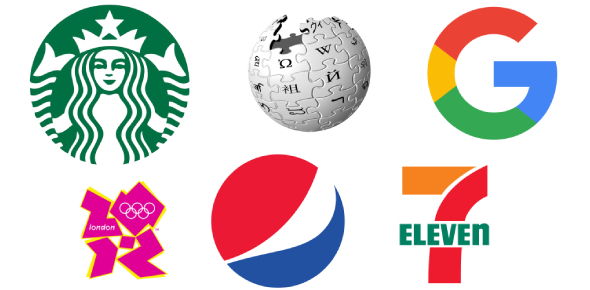# 204 - Decision Science

20 Questions | Attempts: 86
ShareSettings• 1.
Which of the following is a valid objective function for a linear programming problem?
• A.

A) Max 5 XY

• B.

B) Min 4X + 3Y + 6Z

• C.

C) Max 5X2 + 6Y4

• D.

D) Min (X1+X2) / 3

• 2.
Slack is -
• A.

A) the amount by which the left side of the constraint is smaller than the right side

• B.

B) the difference between the left and right sides of a constraint

• C.

C) the amount by which the left side of the constraint is larger than the right side

• D.

D) Exists for each variable in a linear programming problem

• 3.
A solution that satisfies all the constraints of a linear programming problem except the non-negativity constraints is called –
• A.

Optimal

• B.

Feasible

• C.

Infeasible

• D.

Semi-feasible

• 4.
A simulation model uses the mathematical expressions and logical relationships of the –
• A.

Real system

• B.

Computer Model

• C.

Performance Measures

• D.

Estimated inference

• 5.
Analysis of a Markov Process -
• A.

Describes future behavior of the system

• B.

Optimizes the system

• C.

Leads to higher order decision making

• D.

All of the alternatives are true.

• 6.
In a multiple channel system –
• A.

Each server has its own queue

• B.

Each server has the same service rate

• C.

µ > λ

• D.

All of the alternatives are correct

• 7.
The solution of a transportation problem, with m- rows ( supplies) and n – columns ( destinations) is feasible if the number of occupied cells are -
• A.

M + n

• B.

M X n

• C.

M + n -1

• D.

M + n +1

• 8.
The assignment problem -
• A.

Requires that only one activity be assigned to each sequence

• B.

Is a special case of transportation problems

• C.

Can be used for maximization objective

• D.

All of the above.

• 9.
In (M/M/1) : (∞/FCFS) model, the system length  Ls is given by -
• A.

ϱ / 1-ϱ

• B.

λ 2 / µ - λ

• C.

1/ µ - λ

• D.

ρ2 / 1-ρ

• 10.
In the queue model notation (a/b/c) : (d/e), what does c represent –
• A.

Arrival pattern

• B.

Service pattern

• C.

Number of services

• D.

Capacity of the system

• 11.
Customer behavior in which he moves from one queue to another in multiple channel situation is –
• A.

Backing

• B.

Reneging

• C.

Jockeying

• D.

Alternating

• 12.
While improving a solution of transportation problem, we reallocate units to the unoccupied cell having its opportunity cost as –
• A.

Equal to zero

• B.

Most negative number

• C.

Most positive number.

• D.

Any value

• 13.
To balance an Assignment matrix we have to -
• A.

• B.

• C.

Remove a row or column depending upon the given situation

• D.

Add a dummy row or column depending upon a given situation.

• 14.
MODI stands for –
• A.

Modern distributions

• B.

Markov distributions Method

• C.

Modified Distribution Method

• D.

Model Index method

• 15.
The prohibited cell in transportation problem is considered by considering its cost –
• A.

- ∞

• B.

0

• C.

Negative

• D.

• 16.
Assignment problem is solved by –
• A.

Simplex Method

• B.

Graphical Method

• C.

Vector Method

• D.

Hungarian Method

• 17.
The first step in solving operations research problem is –
• A.

Model building

• B.

Obtain alternate solutions

• C.

Interpreting the variables

• D.

Formulation of the problem

• 18.
If Ro represent state probabilities of present period then the state probabilities end of second period is given by –
• A.

R2 = R1 X P2

• B.

R2 = P X R1

• C.

R2 = R0 X P2

• D.

None of the above

• 19.
While using random numbers in Monte Carlo Simulation it is –
• A.

Not necessary to assign the exact range of random numbers

• B.

Necessary to use particular random numbers

• C.

Necessary to find out cumulative probability distribution

• D.

None of the above

• 20.
Utilization factor is –
• A.

Arrival rate divided by service rate

• B.

Service rate divided by arrival rate

• C.

Length in the system divided by length in the queue

• D.

Waiting time in system divided by waiting time in queue

## Related TopicsBack to top
×

Wait!
Here's an interesting quiz for you.